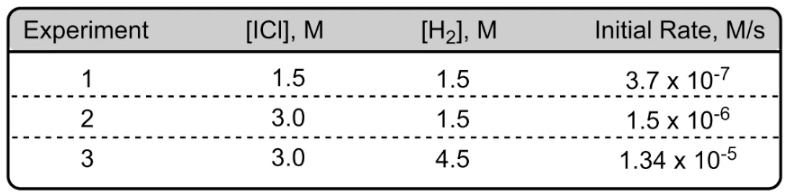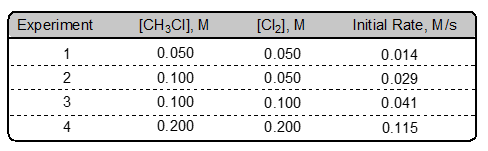Clutch Prep is now a part of Pearson
Ch.13 - Chemical KineticsWorksheetSee all chapters

# Rate Law

See all sections
Sections
Intro to Chemical Kinetics
Energy Diagrams
Catalyst
Factors Influencing Rates
Average Rate of Reaction
Stoichiometric Rate Calculations
Instantaneous Rate
Collision Theory
Arrhenius Equation
Rate Law
Reaction Mechanism
Integrated Rate Law
Half-Life

Rate Law represents an equation for a chemical reaction that connects the reaction rate with the concentrations or pressures of the reactants and the rate constant.

###### Understanding Rate Law

Concept #1: Rate Law

Example #1: The chemical reaction: 2 A + 3 B + C → D has a Rate Law of k[A]2[B][C]0. By what factor would the rate increase if the concentration of A were tripled, the concentration of B was cut by half, the concentration of C increased by half, and the rate constant k was kept constant?

Concept #2: Rate Constant Units

Example #2: What is the overall order and the units for the rate constant k for the following chemical reaction shown below that has Rate = k [NO2]2[Cl2]?

NO2 (g) + Cl2 (g) → NOCl (g) + ClO (g)

Concept #3: Rate Law Determination

Example #3: The initial rates of reaction for 2 NO (g) + Cl2 (g) → 2 NOCl (g) are:

Experiment                   [NO], M                         [Cl2], M                         Initial Rate, M/s

1                  0.0250               0.0510                           18.2

2                  0.0250               0.0255                           9.08

3                 0.0500               0.0255                           18.2

Determine the new rate if given new initial concentrations of [NO] = 0.0730 M and [Cl2] = 0.0510 M.

Practice: Given the following chemical reaction, A → B. If the concentration of A is doubled the rate increases by a factor of 2.83, what is the order of the reaction with respect to A.

Practice: In the experiments on the reaction 2 ICl (g) + H2 (g) → I2 (g) + 2 HCl (g), the following initial rate data were obtained. What is the overall order of the reaction?Practice: The data below were collected for the following reaction: CH3Cl (g) + 3 Cl2 (g) → CCl4 (g) + 3 HCl (g)Calculate the value and units for the rate constant k.

A. 1.25 M–0.5•s–1

B. 5.6 M–1•s–1

C. 25 M3/2•s–1

D. 7.9 M–5/2•s–1